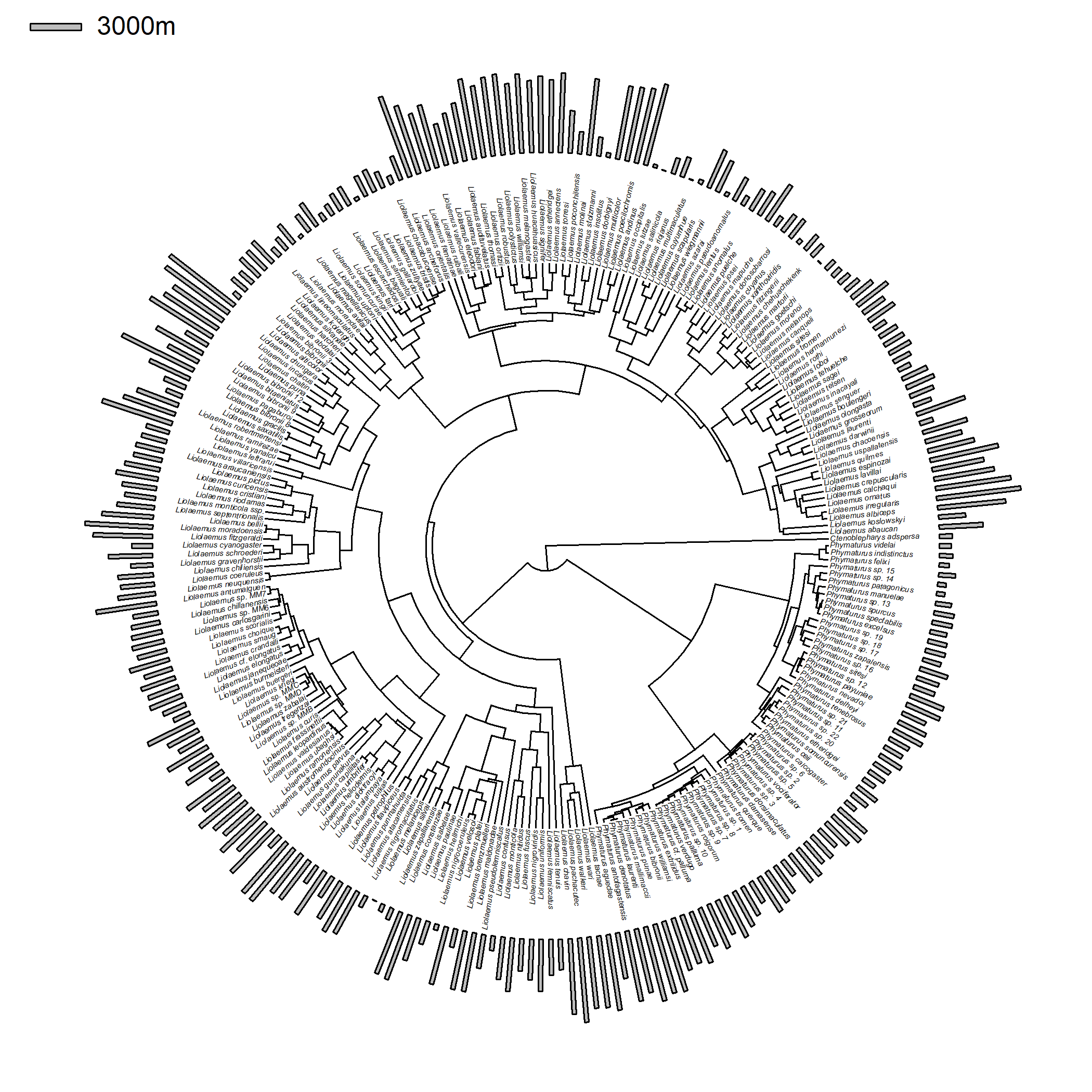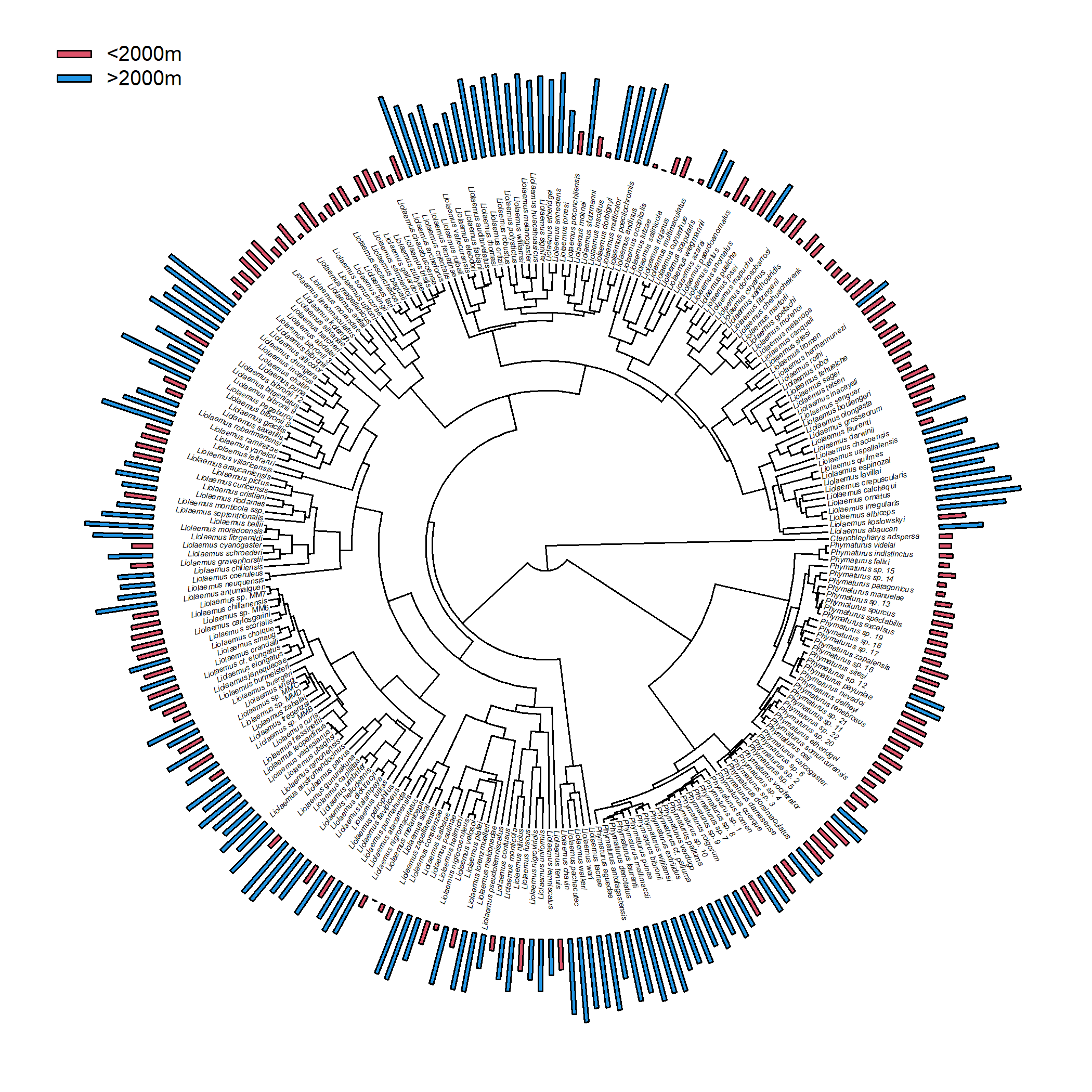## Tuesday, June 14, 2022

### phytools `plotTree.wBars` coloring the bars by our continuous trait

Today, a phytools user writes:

“I am trying to use the command `plotTree.wBars` in phytools to add bars as tips of a circular tree. The bars represent values of a continuous trait assigned to the tips. I was wondering if it is possible to also color these bars according to their values?”

The answer is, of course, yes. Let's see a couple of different examples.

For this example, I'll use a phylogeny and dataset for liolaemid lizards from Esquerre et al. (2019).

Luke Harmon & I use these data in our upcoming book, so the easiest way to get them is from the book website.

``````## load phytools
library(phytools)
liol.tree
``````
``````##
## Phylogenetic tree with 258 tips and 257 internal nodes.
##
## Tip labels:
##   Ctenoblepharys_adspersa, Liolaemus_abaucan, Liolaemus_koslowskyi, Liolaemus_albiceps, Liolaemus_irregularis, Liolaemus_ornatus, ...
##
## Rooted; includes branch lengths.
``````
``````## read data
row.names=1)
``````
``````##                         parity_mode max_altitude temperature
## Liolaemus_abaucan                 O         2600       20.20
## Liolaemus_albiceps                V         4020       12.38
## Liolaemus_andinus                 V         4900       11.40
## Liolaemus_annectens               V         4688        5.10
## Liolaemus_anomalus                O         1400       23.78
``````

I'm going to first plot the tree with `max_altitude` using `plotTree.wBars`. The only trick I'm adding is that I'm going to steal some internal calculations (for `scale=NULL`) to add a scale bar to the tree using `polygon`. If we had set a specific value for `scale`, we'd use that instead of the variable `ss`.

``````max_altitude<-setNames(liol.data\$max_altitude,rownames(liol.data))
plotTree.wBars(liol.tree,max_altitude,type="fan",lwd=1,
tip.label=TRUE,fsize=0.3)
ss<-0.3*max(nodeHeights(liol.tree))/diff(range(max_altitude))
h<-0.95*par()\$usr
bb<-3000
polygon(x=-h+c(0,0,ss*bb,ss*bb),y=h+c(1,0,0,1),col="grey")
text(x=-h+ss*bb,y=h+0.5,paste(bb,"m",sep=""),pos=4)
``````Next, let's do it again, but this time coloring all the bars greater than or equal to 2000m with one color (blue) and all those below 2000m another (red). For this one, I will set the `plotTree.wBars` argument `col` which specifies the fill color of our polygons. The easiest way for me to do this here is by creating a vector `col` containing only the color blue (`palette()` in this case), and then substituting `palette()` for all `max_altitude<2000`.

``````max_altitude<-setNames(liol.data\$max_altitude,rownames(liol.data))
col<-setNames(rep(palette(),length(max_altitude)),names(max_altitude))
col[max_altitude<2000]<-palette()
plotTree.wBars(liol.tree,max_altitude,type="fan",lwd=1,
tip.label=TRUE,fsize=0.3,col=col)
ss<-0.3*max(nodeHeights(liol.tree))/diff(range(max_altitude))
h<-0.9*par()\$usr
bb<-2000
polygon(x=-h+c(0,0,ss*bb,ss*bb),y=h+c(1,0,0,1),col=palette())
text(x=-h+ss*bb,y=h+0.5,paste("<2000m",sep=""),pos=4,cex=0.8)
h<-0.9*par()\$usr
bb<-2000
polygon(x=-h+c(0,0,ss*bb,ss*bb),y=0.95*h+c(1,0,0,1),col=palette())
text(x=-h+ss*bb,y=0.95*h+0.5,paste(">2000m",sep=""),pos=4,cex=0.8)
``````Lastly, let's do it based on a continuous color gradient. I guess this should be the hardest, but I'm pretty sure we can figure it out!

In this case, what I decided to do was to first discretize my continuous character (altitude) by normalizing it to the interval [0,1] and then multiplying this quantity by 1,000 (actually, 999 + 1). After rounding, I have created an index vector between 1 & 1,000. I then create my color ramp with 1,000 colors and pull out those that I want to use in my graph. I chose the `topo.colors` palette, but I could have picked any.

``````ind<-round((max_altitude-min(max_altitude))/diff(range(max_altitude))*999)+1
cols<-setNames(topo.colors(n=1000)[ind],names(ind))
plotTree.wBars(liol.tree,max_altitude,type="fan",lwd=1,
tip.label=TRUE,fsize=0.3,col=cols)
ss<-0.3*max(nodeHeights(liol.tree))/diff(range(max_altitude))
h<-0.9*par()\$usr
bb<-10000